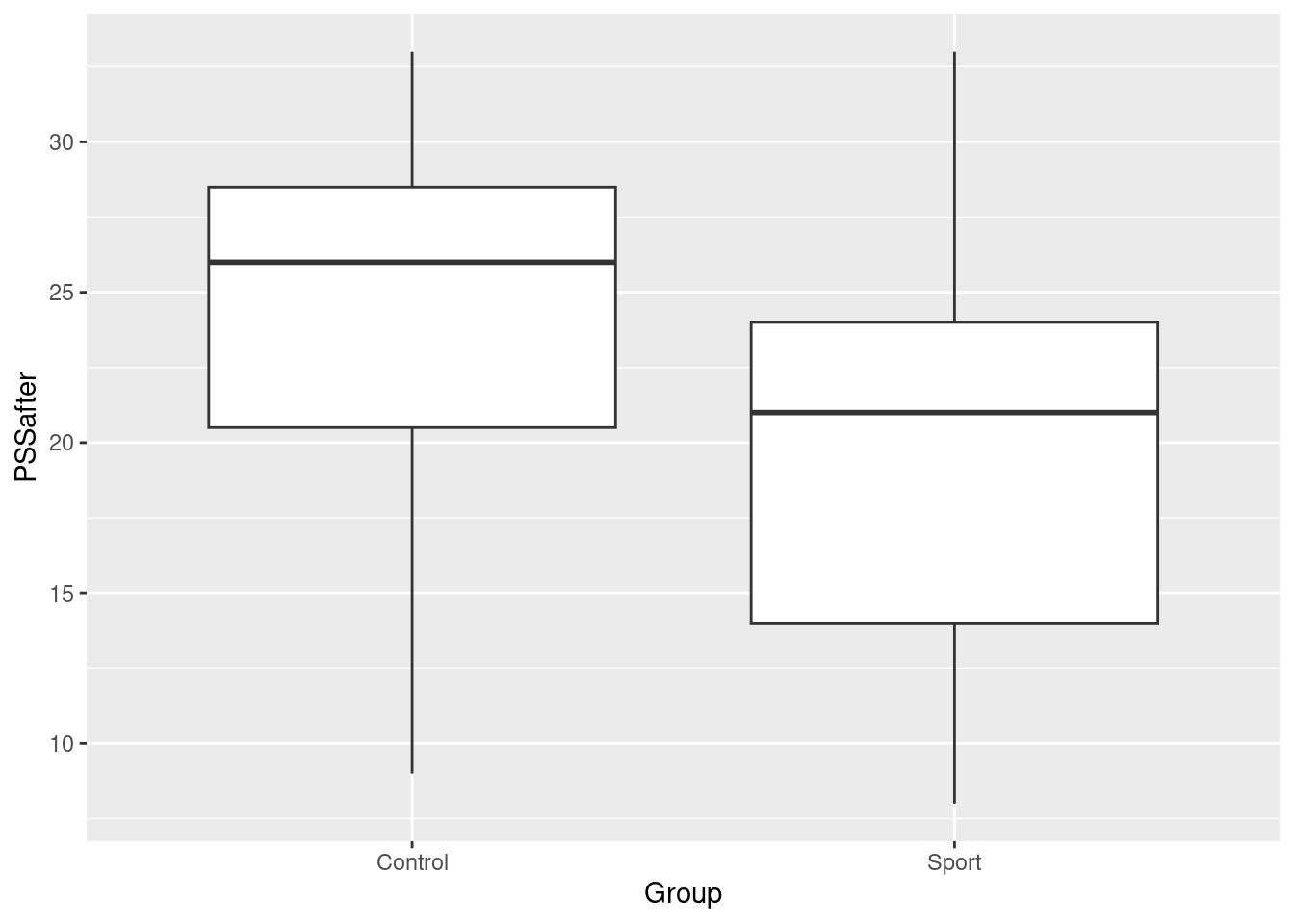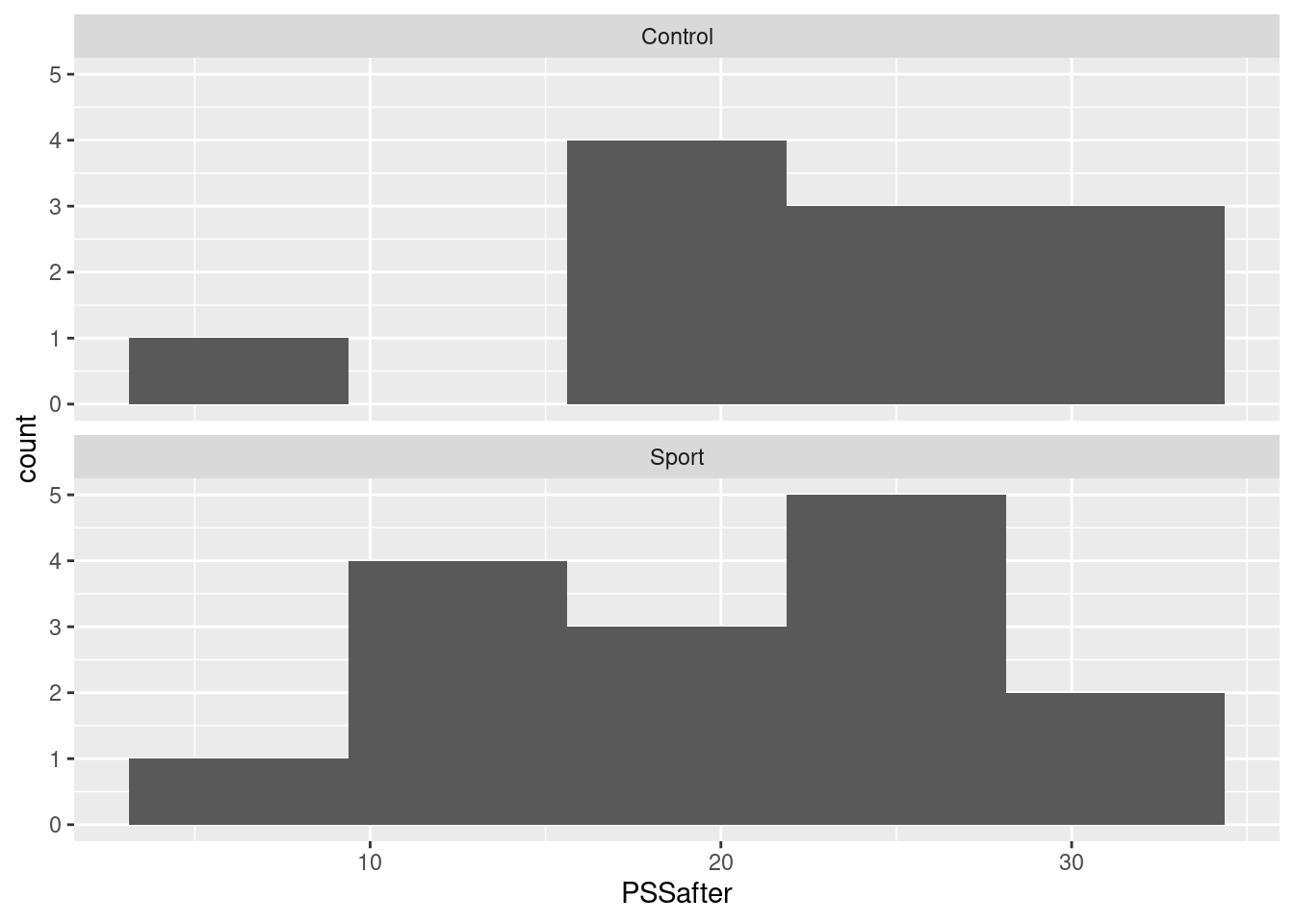# STAC32 Assignment 4

You are expected to complete this assignment on your own: that is, you may discuss general ideas with others, but the writeup of the work must be entirely your own. If your assignment is unreasonably similar to that of another student, you can expect to be asked to explain yourself.

If you run into problems on this assignment, it is up to you to figure out what to do. The only exception is if it is impossible for you to complete this assignment, for example a data file cannot be read. (There is a difference between you not knowing how to do something, which you have to figure out, and something being impossible, which you are allowed to contact me about.)

You must hand in a rendered document that shows your code, the output that the code produces, and your answers to the questions. This should be a file with .html on the end of its name. There is no credit for handing in your unrendered document (ending in .qmd), because the grader cannot then see whether the code in it runs properly. After you have handed in your file, you should be able to see (in Attempts) what file you handed in, and you should make a habit of checking that you did indeed hand in what you intended to, and that it displays as you expect.

Hint: render your document frequently, and solve any problems as they come up, rather than trying to do so at the end (when you may be close to the due date). If your document will not successfully render, it is because of an error in your code that you will have to find and fix. The error message will tell you where the problem is, but it is up to you to sort out what the problem is.

# 1 Prison stress

Being in prison is stressful, for anybody. 26 prisoners took part in a study where their stress was measured at the start and end of the study. Some of the prisoners, chosen at random, completed a physical training program (for these prisoners, the Group column is Sport) and some did not (Group is Control). The researchers’ main aim was to see whether the physical training program reduced stress on average in the population of prisoners. The data are in http://www.ritsokiguess.site/datafiles/PrisonStress.csv, in four columns, respectively an identifier for the prisoner, whether or not they did physical training, their stress score at the start of the study, and their stress score at the end.

## (a) (2 points) Read in and display (some of) the data.

Very much the usual. Give the dataframe a name of your choosing; stress is good as a name because none of the columns are actually called stress:

my_url <- "http://www.ritsokiguess.site/datafiles/PrisonStress.csv"
stress <- read_csv(my_url)
Rows: 26 Columns: 4
── Column specification ────────────────────────────────────────────────────────
Delimiter: ","
chr (2): Subject, Group
dbl (2): PSSbefore, PSSafter

ℹ Use spec() to retrieve the full column specification for this data.
ℹ Specify the column types or set show_col_types = FALSE to quiet this message.
stress

As you see, the columns of stress scores are called PSSbefore and PSSafter, which is because PSS was the name of the stress scale the researchers used.

## (b) (2 points) Make a suitable graph of the stress scores at the end of the study and whether or not each prisoner was in the Sport group.

These are the columns PSSafter (quantitative) and Group (categorical), so as is the way with these things, you need a boxplot:

ggplot(stress, aes(x = Group, y = PSSafter)) + geom_boxplot()Unlike the one on the midterm, you don’t need to make the boxes go left and right (although no harm if you do). A vertical boxplot is fine here.

An alternative is to reason that you have one quantitative variable (PSSafter) and “too many” categorical variables (Group), so you do histograms facetted by Group:

ggplot(stress, aes(x = PSSafter)) + geom_histogram(bins = 5) +
facet_wrap(~ Group, ncol = 1)choosing a suitable number of bins (I think about 6 bins is as high as you want to go).

## (c) (3 points) Run the most appropriate $$t$$-test to compare the stress scores at the end of the study for the two groups of prisoners. Bear in mind what the researchers are trying to show. What do you conclude from your test, in the context of the data?

This means comparing PSSafter between the two groups defined in Group. The researchers wanted to show that the average (mean) stress score is lower for the prisoners who did the physical training, so we need a one-sided test. The two groups in Group are Sport and Control; the second of these is first alphabetically, so to get the right test we need to say how Control compares to Sport in that (alphabetical) order.

The other thing to consider is whether we should be doing a Welch or a pooled test. I’m prepared to entertain either of these, as long as you state a reason for doing the one you do. My take is that the two groups differ slightly in spread (the boxes on the boxplots differ slightly in height), so I would do the Welch test:

t.test(PSSafter ~ Group, data = stress,
alternative = "greater")

Welch Two Sample t-test

data:  PSSafter by Group
t = 1.3361, df = 21.325, p-value = 0.09781
alternative hypothesis: true difference in means between group Control and group Sport is greater than 0
95 percent confidence interval:
-1.069768       Inf
sample estimates:
mean in group Control   mean in group Sport
23.72727              20.00000 

This gives a P-value of 0.09781, which is not smaller than 0.05, so I cannot reject the null hypothesis, and so there is no evidence here that the physical training reduces stress on average in prisoners.

I think you could also reasonably say that the two groups do not differ substantially in spread, on the basis that the boxes on the boxplot are not very different in height, and therefore that the pooled test would also work:

t.test(PSSafter ~ Group, data = stress,
var.equal = TRUE, alternative = "greater")

Two Sample t-test

data:  PSSafter by Group
t = 1.3424, df = 24, p-value = 0.09601
alternative hypothesis: true difference in means between group Control and group Sport is greater than 0
95 percent confidence interval:
-1.023091       Inf
sample estimates:
mean in group Control   mean in group Sport
23.72727              20.00000 

The P-value is almost identical, and the conclusion is the same, that there is no evidence that the physical training is effective in reducing stress.

The question to ask yourself, when looking over this afterwards, is therefore not “did I do the right test?”, but instead “did I do the test I did for a good reason?”.

## (d) (3 points) Make a suitable plot of the stress measurements before the study for each group of prisoners. How, if at all, does that impact the conclusion you drew in the previous part? Explain briefly.

This is another boxplot, which is not in itself very exciting, but what is interesting is the conclusion it helps us to draw. (Therefore, one point only for the boxplot, and two for the conclusion this time):

ggplot(stress, aes(x = Group, y = PSSbefore)) +
geom_boxplot()